# reflector

Create reflector-backed antenna

## Description

The `reflector` object is a reflector-backed antenna on the xyz- plane. The default reflector antenna uses a dipole as an exciter. The feed point is on the exciter.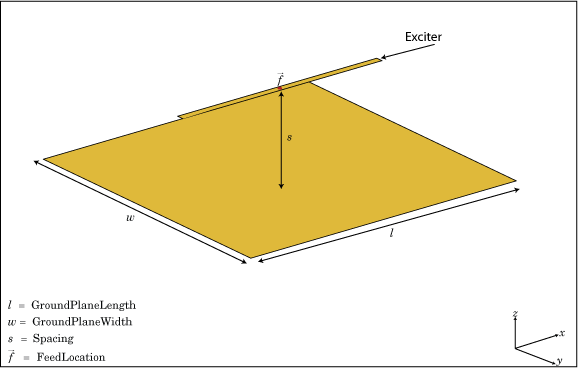## Creation

### Syntax

``rf = reflector``
``rf = reflector(Name=Value)``

### Description

``` `rf = reflector` creates a reflector backed antenna located in the X-Y-Z plane. By default, dimensions are chosen for an operating frequency of 1 GHz.```

example

``` `rf = reflector(Name=Value)` creates a reflector antenna, with additional properties specified by one or more name-value arguments. `Name` is the property name and `Value` is the corresponding value. You can specify several name-value arguments in any order as `Name1`=`Value1`, `...`, `NameN`=`ValueN`. Properties not specified retain their default values.For example, `r = reflector(Exciter=yagiUda)` creates a reflector antenna with a Yagi Uda as an exciter.```

## Properties

expand all

Exciter antenna or array type, specified as a single-element antenna object, an array object, or an empty array. Except for reflector and cavity antenna elements, you can use any Antenna Toolbox™ antenna or array element as an exciter. To create the cavity backing structure without the exciter, specify this property as an empty array.

Example: `horn`

Example: `linearArray(Element=patchMicrostrip)`

Example: `[]`

Substrate dielectric material, specified as "air" or a `dielectric` object. For more information about dielectric substrate meshing, see Meshing.

Note

The substrate dimensions must be equal to the groundplane dimensions.

Example: `dielectric("FR4")`

Reflector length along the x-axis, specified a scalar in meters. By default, ground plane length is measured along the x-axis. Setting `GroundPlaneLength` to`Inf`, uses the infinite ground plane technique for antenna analysis. You can also set the `GroundPlaneLength` to zero.

Example: `3`

Data Types: `double`

Reflector width along the y-axis, specified as a scalar in meters. By default, ground plane width is measured along the y-axis. Setting `GroundPlaneWidth` to`Inf`, uses the infinite ground plane technique for antenna analysis. You can also set the `GroundPlaneWidth` to zero.

Example: `2.5`

Data Types: `double`

Distance between the reflector and the exciter, specified as a scalar in meters. By default, the exciter is placed along the x-axis.

Example: `7.5e-2`

Data Types: `double`

Type of the metal used as a conductor, specified as a metal material object. You can choose any metal from the `MetalCatalog` or specify a metal of your choice. For more information, see `metal`. For more information on metal conductor meshing, see Meshing.

Example: `metal("Copper")`

Lumped elements added to the antenna feed, specified as a lumped element object. For more information, see `lumpedElement`.

Example: `Load=lumpedelement`. `lumpedelement` is the object for the load created using `lumpedElement`.

Example: `lumpedElement(Impedance=75)`

Create probe feed from backing structure to exciter, specified as `0` (disable) or `1` (enable). By default, probe feed is disabled.

Example: `1`

Data Types: `double` | `logical`

Tilt angle of the antenna in degrees, specified as a scalar or vector. For more information, see Rotate Antennas and Arrays.

Example: `90`

Example: `Tilt=[90 90]`,`TiltAxis=[0 1 0;0 1 1]` tilts the antenna at 90 degrees about the two axes defined by the vectors.

Data Types: `double`

Tilt axis of the antenna, specified as one of these values:

• Three-element vector of Cartesian coordinates in meters. In this case, each coordinate in the vector starts at the origin and lies along the specified points on the x-, y-, and z-axes.

• Two points in space, specified as a 2-by-3 matrix corresponding to two three-element vectors of Cartesian coordinates. In this case, the antenna rotates around the line joining the two points.

• `"x"`, `"y"`, or `"z"` to describe a rotation about the x-, y-, or z-axis, respectively.

Example: `[0 1 0]`

Example: `[0 0 0;0 1 0]`

Example: `"Z"`

Data Types: `double` | `string`

## Object Functions

 `show` Display antenna, array structures, shapes, or platform `info` Display information about antenna or array `axialRatio` Axial ratio of antenna `beamwidth` Beamwidth of antenna `charge` Charge distribution on antenna or array surface `current` Current distribution on antenna or array surface `design` Design prototype antenna or arrays for resonance around specified frequency or create AI-based antenna from antenna catalog objects `efficiency` Radiation efficiency of antenna `EHfields` Electric and magnetic fields of antennas or embedded electric and magnetic fields of antenna element in arrays `impedance` Input impedance of antenna or scan impedance of array `mesh` Mesh properties of metal, dielectric antenna, or array structure `meshconfig` Change meshing mode of antenna, array, custom antenna, custom array, or custom geometry `optimize` Optimize antenna or array using SADEA optimizer `pattern` Plot radiation pattern and phase of antenna or array or embedded pattern of antenna element in array `patternAzimuth` Azimuth plane radiation pattern of antenna or array `patternElevation` Elevation plane radiation pattern of antenna or array `rcs` Calculate and plot radar cross section (RCS) of platform, antenna, or array `returnLoss` Return loss of antenna or scan return loss of array `sparameters` Calculate S-parameters for antennas and antenna arrays `vswr` Voltage standing wave ratio (VSWR) of antenna or array element

## Examples

collapse all

Create a reflector backed dipole that has 30 cm length, 25 cm width and spaced 7.5 cm from the dipole for operation at 1 GHz.

```d = dipole(Length=0.15,Width=0.015,Tilt=90,TiltAxis=[0 1 0]); rf = reflector(GroundPlaneLength=30e-2,GroundPlaneWidth=25e-2,... Spacing=7.5e-2,Exciter=d); show(rf)```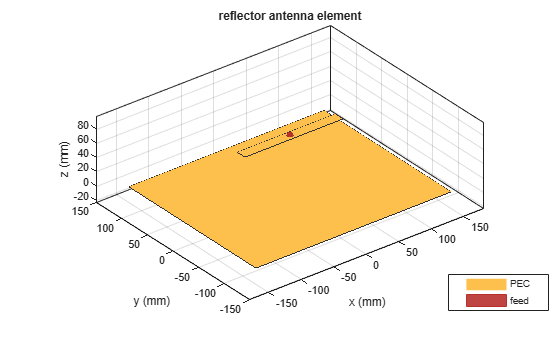Create a reflector backed dipole antenna using a FR4 dielectric substrate.

```d = dielectric("FR4"); di = dipole(Length=0.15,Width=0.015,Tilt=90,TiltAxis='Y'); rf = reflector(GroundPlaneLength=30e-2,GroundPlaneWidth=25e-2,... Spacing=7.5e-3,Substrate=d,Exciter=di); show(rf)```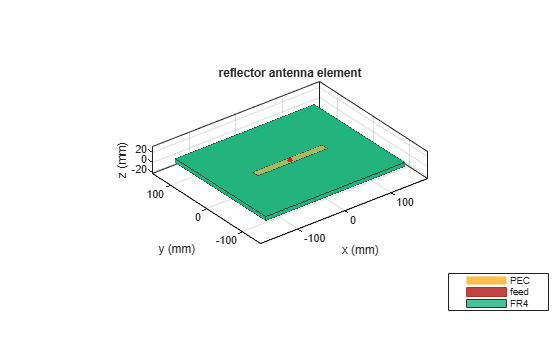Plot the radiation pattern of the antenna at a frequency of 1 GHz.

```figure pattern(rf,1e9)```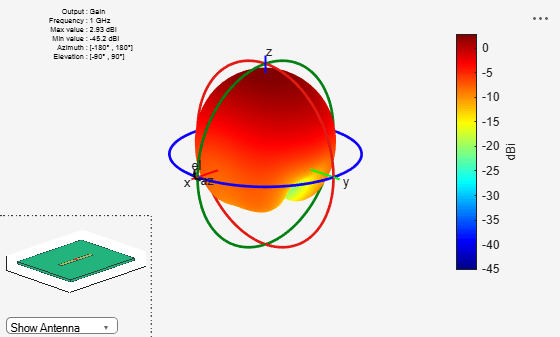Create a reflector backed dipole that has infinite length, 25 cm width and spaced 7.5 cm from the dipole for operation at 1 GHz.

```d = dipole(Length=0.15,Width=0.015,Tilt=90,TiltAxis=[0 1 0]); rf = reflector(GroundPlaneLength=inf,GroundPlaneWidth=25e-2,... Spacing=7.5e-2,Exciter=d)```
```rf = reflector with properties: Exciter: [1x1 dipole] Substrate: [1x1 dielectric] GroundPlaneLength: Inf GroundPlaneWidth: 0.2500 Spacing: 0.0750 EnableProbeFeed: 0 Conductor: [1x1 metal] Tilt: 0 TiltAxis: [1 0 0] Load: [1x1 lumpedElement] ```
`show(rf)`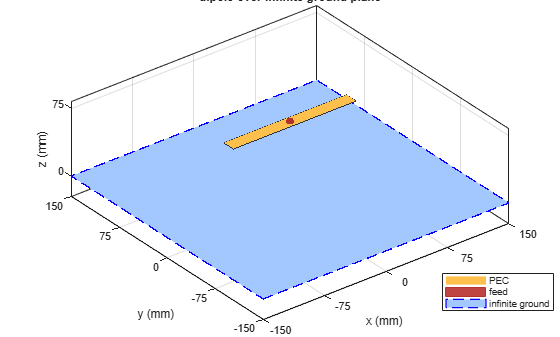Compare the gain values of a dipole antenna in free space and dipole antenna on a substrate.

Design a dipole antenna at a frequency of 1 GHz.

```d = design(dipole,1e9); l_by_w = d.Length/d.Width; d.Tilt = 90; d.TiltAxis = [0 1 0];```

Plot the radiation pattern of the dipole in free space at 1 GHz.

```figure pattern(d,1e9);```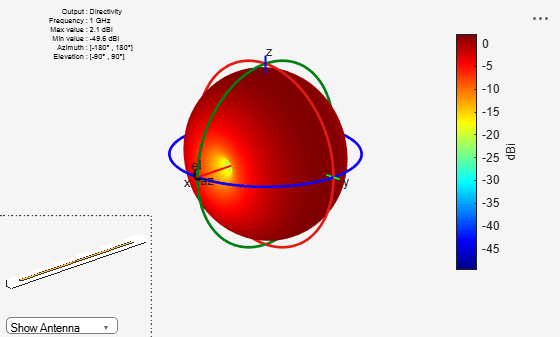Use FR4 as the dielectric substrate.

`t = dielectric("FR4")`
```t = dielectric with properties: Name: 'FR4' EpsilonR: 4.8000 LossTangent: 0.0260 Thickness: 0.0060 For more materials see catalog ```
```eps_r = t.EpsilonR; lambda_0 = physconst("lightspeed")/1e9; lambda_d = lambda_0/sqrt(eps_r);```

Adjust the length of the dipole based on the wavelength.

```d.Length = lambda_d/2; d.Width = d.Length/l_by_w;```

Design a reflector at 1 GHz with the dipole as the exciter and FR4 as the substrate.

```rf = reflector(Exciter=d,Spacing=7.5e-3,Substrate=t); rf.GroundPlaneLength = lambda_d; rf.GroundPlaneWidth = lambda_d/4; figure show(rf)```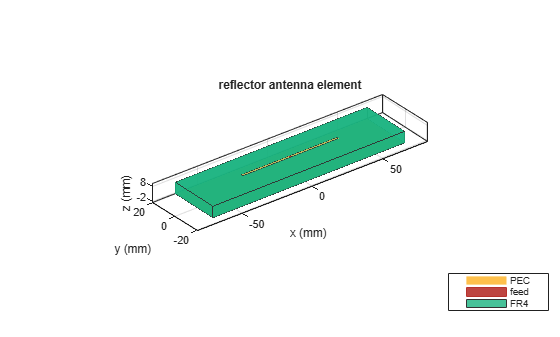Remove the groundplane for plotting the gain of the dipole on the substrate.

```rf.GroundPlaneLength = 0; show(rf)```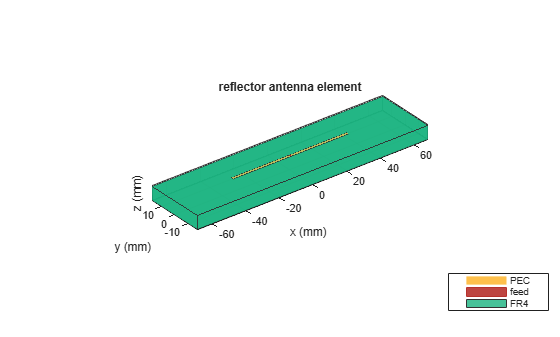Plot the radiation pattern of the dipole on the substrate at 1 GHz.

```figure pattern(rf,1e9);```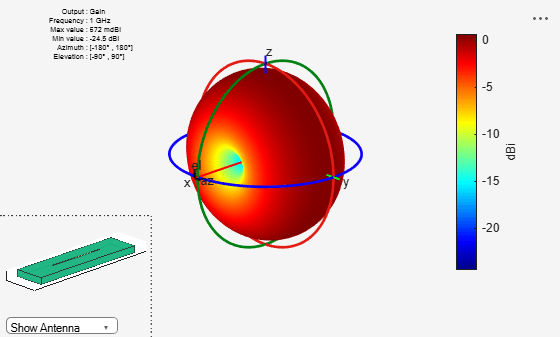Compare the gain values.

• Gain of the dipole in free space = 2.11 dBi

• Gain of the dipole on substrate = 1.93 dBi

Create a rectangular array of the bowtie antennas.

`b = bowtieTriangular(Length=0.05)`
```b = bowtieTriangular with properties: Length: 0.0500 FlareAngle: 90 Conductor: [1x1 metal] Tilt: 0 TiltAxis: [1 0 0] Load: [1x1 lumpedElement] ```
`rectArr = rectangularArray(Element=b,RowSpacing=0.18,ColumnSpacing=0.18)`
```rectArr = rectangularArray with properties: Element: [1x1 bowtieTriangular] Size: [2 2] RowSpacing: 0.1800 ColumnSpacing: 0.1800 Lattice: 'Rectangular' AmplitudeTaper: 1 PhaseShift: 0 Tilt: 0 TiltAxis: [1 0 0] ```

Create a rectangular array with reflector backing structure.

`ant = reflector(Exciter=rectArr)`
```ant = reflector with properties: Exciter: [1x1 rectangularArray] Substrate: [1x1 dielectric] GroundPlaneLength: 0.2000 GroundPlaneWidth: 0.2000 Spacing: 0.0750 EnableProbeFeed: 0 Conductor: [1x1 metal] Tilt: 0 TiltAxis: [1 0 0] Load: [1x1 lumpedElement] ```
`show(ant)`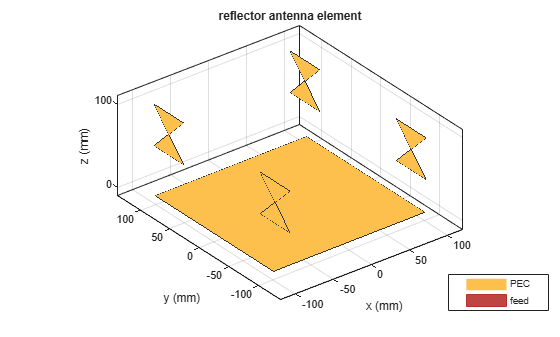Create a reflector-backed rectangular array of microstrip patch antennas.

```p = patchMicrostrip(Substrate=dielectric("FR4")); ra = rectangularArray(Element=p,RowSpacing=0.075,ColumnSpacing=0.1); ant = reflector(Exciter=ra,GroundPlaneLength=0.4,GroundPlaneWidth=0.3)```
```ant = reflector with properties: Exciter: [1x1 rectangularArray] Substrate: [1x1 dielectric] GroundPlaneLength: 0.4000 GroundPlaneWidth: 0.3000 Spacing: 0.0750 EnableProbeFeed: 0 Conductor: [1x1 metal] Tilt: 0 TiltAxis: [1 0 0] Load: [1x1 lumpedElement] ```
`show(ant)`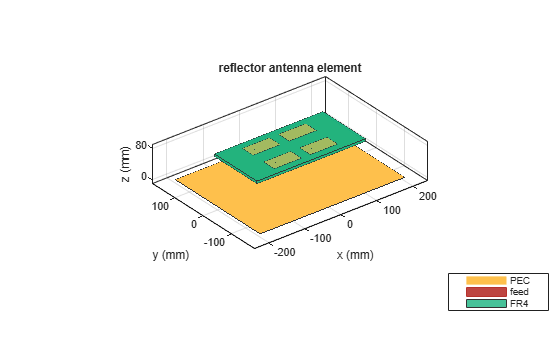Balanis, C.A. Antenna Theory. Analysis and Design, 3rd Ed. New York: Wiley, 2005.